Related Articles
Spatial Segregation in Social Networks
• Last Updated : 01 Oct, 2020

Prerequisite – Introduction to Social Networks

Segregation refers to the act of separation of people from others or the main group.

Spatial Segregation refers to the distribution of social groups or any other elements in space. In Spatial Segregation, people tend to migrate to other places where they have more neighbors who are like them.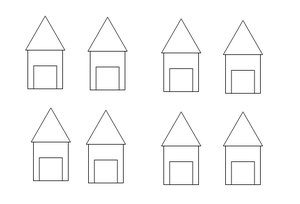Group  of Houses in a locality

According to spatial segregation, it is unlikely that a person can stay at a place where the neighbors are very different from you and you cannot have a good conversation with your neighbor. A person will more likely to migrate to a place where his/her neighbors are similar to them and you can have a good conversation with your neighbor.

Let’s take an example – Assume you went to a foreign country for living and you are deciding where to take the house. So you will more likely to choose a place or locality where your neighbors are from your country and speaks the same language as yours and not a place where people are from a different country and speaks a different language. This is spatial segregation.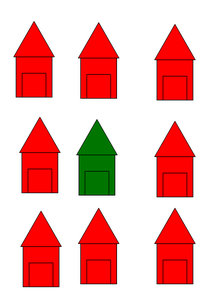Locality with all different neighbors from you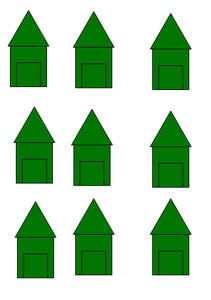Locality with all same neighbors as you

But sometimes it is not possible to have all neighbors as same as you. So for that, we can have some threshold value for similar neighbors to stay there.

Let’s take an example – Assume there is a total of 9 houses in your locality including yours and let’s say there is a threshold of 3 which means that at least 3 houses should be similar to you.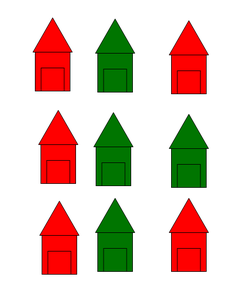The person will stay here as t =3

This spatial segregation has been modeled and this model is known as the Schelling Model. In the Schelling model, there is a grid having 2 different types of people and simulation describes how spatial segregation has been done using a wonderful model. Below is the implementation of such a simulation in Python.

Example:

A grid of 10×10 dimension is created for demonstration. Consider three groups of nodes which are differentiated with the colors red, green and white. Now Based on the Schelling model the nodes are moved towards the nodes similar to it. Here the similarity is based on the color. More the number of iterations, Less the number of unlike neighbors.

## Python3

 `#import modules``import` `networkx as nx``import` `matplotlib.pyplot as plt``import` `random`` ` `# grid dimension``n ``=` `10`` ` `# Building the graph``g ``=` `nx.grid_2d_graph(``10``, ``10``)``pos ``=` `dict``((s, s) ``for` `s ``in` `g.nodes())``labels ``=` `dict``(((i, j), i ``*` `10` `+` `j) ``for` `i, j ``in` `g.nodes())`` ` `# Display the graph in grid form.``def` `display(g):``    ``nodes_g ``=` `nx.draw_networkx_nodes(``        ``g, pos, node_color``=``'green'``, nodelist``=``type1_node_list)``    ``nodes_r ``=` `nx.draw_networkx_nodes(``        ``g, pos, node_color``=``'red'``, nodelist``=``type2_node_list)``    ``nodes_w ``=` `nx.draw_networkx_nodes(``        ``g, pos, node_color``=``'white'``, nodelist``=``empty_cells)``    ``nx.draw_networkx_edges(g, pos)``    ``nx.draw_networkx_labels(g, pos, labels``=``labels)``    ``plt.show()`` ` ` ` `# Get the boundary nodes in the graph as boundary nodes have only 6 neighbors.``def` `get_boundary(g):``    ``boundary_nodes_list ``=` `[]``    ``for` `((u, v), d) ``in` `g.nodes(data``=``True``):``        ``if` `(u ``=``=` `0` `or` `v ``=``=` `n ``-` `1` `or` `u ``=``=` `n ``-` `1` `or` `v ``=``=` `0``):``            ``boundary_nodes_list.append((u, v))``    ``return` `boundary_nodes_list`` ` ` ` `# Get internal neighbors.``def` `get_internal_neigh(u, v):``    ``return` `(``        ``[(u ``-` `1``, v), (u ``+` `1``, v), (u, v ``-` `1``), (u, v ``+` `1``), (u ``-` `1``, v ``+` `1``),``         ``(u ``+` `1``, v ``-` `1``), (u ``-` `1``, v ``-` `1``), (u ``+` `1``, v ``+` `1``)])`` ` ` ` `# Get boundary neighbors.``def` `get_boundary_neigh(u, v):``    ``if` `(u ``=``=` `0` `and` `v ``=``=` `0``):``        ``return` `([(``0``, ``1``), (``1``, ``1``), (``1``, ``0``)])``    ``elif` `(u ``=``=` `n ``-` `1` `and` `v ``=``=` `n ``-` `1``):``        ``return` `([(n ``-` `2``, n ``-` `2``), (n ``-` `1``, n ``-` `2``), (n ``-` `2``, n ``-` `1``)])``    ``elif` `(u ``=``=` `n ``-` `1` `and` `v ``=``=` `0``):``        ``return` `([(u ``-` `1``, v), (u, v ``+` `1``), (u ``-` `1``, v ``+` `1``)])``    ``elif` `(u ``=``=` `0` `and` `v ``=``=` `n ``-` `1``):``        ``return` `([(u ``+` `1``, v), (u ``+` `1``, v ``-` `1``), (u, v ``-` `1``)])``    ``elif` `(u ``=``=` `0``):``        ``return` `([(u, v ``-` `1``), (u, v ``+` `1``), (u ``+` `1``, v), (u ``+` `1``, v ``-` `1``), (u ``+` `1``, v ``+` `1``)])``    ``elif` `(v ``=``=` `n ``-` `1``):``        ``return` `([(u, v ``-` `1``), (u ``-` `1``, v), (u ``+` `1``, v), (u ``-` `1``, v ``-` `1``), (u ``+` `1``, v ``-` `1``)])``    ``elif` `(u ``=``=` `n ``-` `1``):``        ``return` `([(u ``-` `1``, v), (u, v ``-` `1``), (u, v ``+` `1``), (u ``-` `1``, v ``+` `1``), (u ``-` `1``, v ``-` `1``)])``    ``elif` `(v ``=``=` `0``):``        ``return` `([(u ``-` `1``, v), (u ``+` `1``, v), (u, v ``+` `1``), (u ``-` `1``, v ``+` `1``), (u ``+` `1``, v ``+` `1``)])`` ` ` ` `# Get the list of unsatisfied nodes in the graph.``def` `get_unsatisfied_nodes_list(g, internal_nodes_list, boundary_nodes_list):``    ``unsatisfied_nodes_list ``=` `[]``    ``t ``=` `3``    ``for` `u, v ``in` `g.nodes():``        ``type_of_this_node ``=` `g.nodes[(u, v)][``'type'``]`` ` `        ``if` `(type_of_this_node ``=``=` `0``):``            ``continue``        ``else``:``            ``similar_nodes ``=` `0``            ``if` `((u, v) ``in` `internal_nodes_list):``                ``neigh ``=` `get_internal_neigh(u, v)``            ``elif` `((u, v) ``in` `boundary_nodes_list):``                ``neigh ``=` `get_boundary_neigh(u, v)`` ` `            ``for` `each ``in` `neigh:``                ``if` `(g.nodes[each][``'type'``] ``=``=` `type_of_this_node):``                    ``similar_nodes ``+``=` `1``            ``if` `(similar_nodes <``=` `t):``                ``unsatisfied_nodes_list.append((u, v))`` ` `    ``return` `unsatisfied_nodes_list`` ` ` ` `# Make the node satisfied by shifting the position``# of unsatisfied node randomly and check if it becomes satisfied.``def` `make_a_node_satisfied(unsatisfied_nodes_list, empty_cells):``    ``if` `(``len``(unsatisfied_nodes_list) !``=` `0``):``        ``node_to_shift ``=` `random.choice(unsatisfied_nodes_list)``        ``new_position ``=` `random.choice(empty_cells)`` ` `        ``g.nodes[new_position][``'type'``] ``=` `g.nodes[node_to_shift][``'type'``]``        ``g.nodes[node_to_shift][``'type'``] ``=` `0``        ``labels[node_to_shift], labels[new_position] ``=` `labels[new_position],``        ``labels[node_to_shift]``    ``else``:``        ``pass`` ` ` ` `# for adding diagonal edges``for` `(u, v) ``in` `g.nodes():``    ``if` `(u ``+` `1` `<``=` `n ``-` `1` `and` `v ``+` `1` `<``=` `n ``-` `1``):``        ``g.add_edge((u, v), (u ``+` `1``, v ``+` `1``))``    ``if` `(u ``+` `1` `<``=` `n ``-` `1` `and` `v ``-` `1` `>``=` `0``):``        ``g.add_edge((u, v), (u ``+` `1``, v ``-` `1``))`` ` ` ` `for` `ni ``in` `g.nodes():``    ``g.nodes[ni][``'type'``] ``=` `random.randint(``0``, ``2``)`` ` `type1_node_list ``=` `[ni ``for` `(ni, d) ``in` `g.nodes(data``=``True``) ``if` `d[``'type'``] ``=``=` `1``]``type2_node_list ``=` `[ni ``for` `(ni, d) ``in` `g.nodes(data``=``True``) ``if` `d[``'type'``] ``=``=` `2``]``empty_cells ``=` `[ni ``for` `(ni, d) ``in` `g.nodes(data``=``True``) ``if` `d[``'type'``] ``=``=` `0``]``display(g)`` ` `boundary_nodes_list ``=` `get_boundary(g)``internal_nodes_list ``=` `list``(``set``(g.nodes()) ``-` `set``(boundary_nodes_list))`` ` `unsatisfied_nodes_list ``=` `get_unsatisfied_nodes_list(g, internal_nodes_list,``                                                    ``boundary_nodes_list)`` ` ` ` `# Loop to move unsatisfied nodes``# vary the value of for loop to visualize different results``for` `i ``in` `range``(``10000``):``    ``unsatisfied_nodes_list ``=` `get_unsatisfied_nodes_list(g, internal_nodes_list,``                                                        ``boundary_nodes_list)`` ` `    ``make_a_node_satisfied(unsatisfied_nodes_list, empty_cells)`` ` `    ``type1_node_list ``=` `[ni ``for` `(ni, d) ``in` `g.nodes(data``=``True``) ``if` `d[``'type'``] ``=``=` `1``]``    ``type2_node_list ``=` `[ni ``for` `(ni, d) ``in` `g.nodes(data``=``True``) ``if` `d[``'type'``] ``=``=` `2``]``    ``empty_cells ``=` `[ni ``for` `(ni, d) ``in` `g.nodes(data``=``True``) ``if` `d[``'type'``] ``=``=` `0``]`` ` `display(g)`

Output: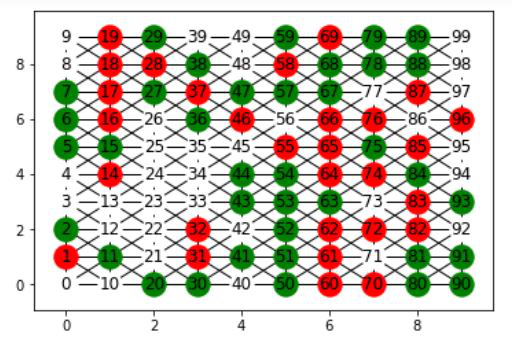initial graph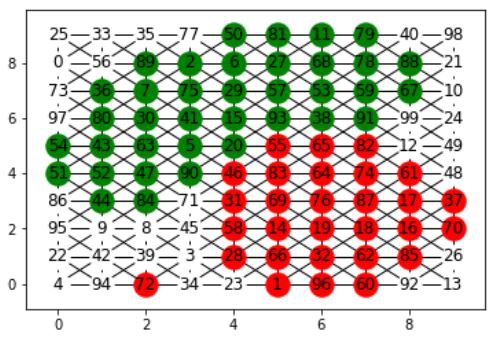After 10,000 iterations

Attention geek! Strengthen your foundations with the Python Programming Foundation Course and learn the basics.

To begin with, your interview preparations Enhance your Data Structures concepts with the Python DS Course. And to begin with your Machine Learning Journey, join the Machine Learning – Basic Level Course

My Personal Notes arrow_drop_up